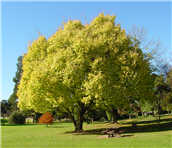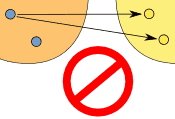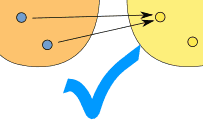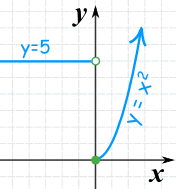# What is a Function?

A function relates an input to an output.It is like a machine that has an input and an output.

And the output is related somehow to the input.

 f(x) "f(x) = ... " is the classic way of writing a function. And there are other ways, as you will see!

## Input, Relationship, Output

We will see many ways to think about functions, but there are always three main parts:

• The input
• The relationship
• The output

### Example: "Multiply by 2" is a very simple function.

Here are the three parts:

Input Relationship Output
0 × 2 0
1 × 2 2
7 × 2 14
10 × 2 20
... ... ...

For an input of 50, what is the output?

## Some Examples of Functions

• x2 (squaring) is a function
• x3+1 is also a function
• Sine, Cosine and Tangent are functions used in trigonometry
• and there are lots more!

But we are not going to look at specific functions ...
... instead we will look at the general idea of a function.

## Names

First, it is useful to give a function a name.

The most common name is "f", but we can have other names like "g" ... or even "marmalade" if we want.

But let's use "f":We say "f of x equals x squared"

what goes into the function is put inside parentheses () after the name of the function:

So f(x) shows us the function is called "f", and "x" goes in

And we usually see what a function does with the input:

f(x) = x2 shows us that function "f" takes "x" and squares it.

Example: with f(x) = x2:

• an input of 4
• becomes an output of 16.

In fact we can write f(4) = 16.

## The "x" is Just a Place-Holder!

Don't get too concerned about "x", it is just there to show us where the input goes and what happens to it.

It could be anything!

So this function:

f(x) = 1 - x + x2

Is the same function as:

• f(q) = 1 - q + q2
• h(A) = 1 - A + A2
• w(θ) = 1 - θ + θ2

The variable (x, q, A, etc) is just there so we know where to put the values:

f(2) = 1 - 2 + 22 = 3

## Sometimes There is No Function Name

Sometimes a function has no name, and we see something like:

y = x2

But there is still:

• an input (x)
• a relationship (squaring)
• and an output (y)

## Relating

At the top we said that a function was like a machine. But a function doesn't really have belts or cogs or any moving parts - and it doesn't actually destroy what we put into it!

A function relates an input to an output.

Saying "f(4) = 16" is like saying 4 is somehow related to 16. Or 4 → 16Example: this tree grows 20 cm every year, so the height of the tree is related to its age using the function h:

h(age) = age × 20

So, if the age is 10 years, the height is:

h(10) = 10 × 20 = 200 cm

Here are some example values:

age h(age) = age × 20
0 0
1 20
3.2 64
15 300
... ...

## What Types of Things Do Functions Process?

"Numbers" seems an obvious answer, but ...

 ... which numbers? For example, the tree-height function h(age) = age×20 makes no sense for an age less than zero. ... it could also be letters ("A"→"B"), or ID codes ("A6309"→"Pass") or stranger things.

So we need something more powerful, and that is where sets come in:### A set is a collection of things.

Here are some examples:

• Set of even numbers: {..., -4, -2, 0, 2, 4, ...}
• Set of clothes: {"hat","shirt",...}
• Set of prime numbers: {2, 3, 5, 7, 11, 13, 17, ...}
• Positive multiples of 3 that are less than 10: {3, 6, 9}

Each individual thing in the set (such as "4" or "hat") is called a member, or element.

So, a function takes elements of a set, and gives back elements of a set.

## A Function is Special

But a function has special rules:

• It must work for every possible input value
• And it has only one relationship for each input value

This can be said in one definition:### Formal Definition of a Function

A function relates each element of a set
with exactly one element of another set
(possibly the same set).

## The Two Important Things!

 1 "...each element..." means that every element in X is related to some element in Y. We say that the function covers X (relates every element of it). (But some elements of Y might not be related to at all, which is fine.)
 2 "...exactly one..." means that a function is single valued. It will not give back 2 or more results for the same input. So "f(2) = 7 or 9" is not right!
 "One-to-many" is not allowed, but "many-to-one" is allowed:(one-to-many) (many-to-one) This is NOT OK in a function But this is OK in a function

When a relationship does not follow those two rules then it is not a function ... it is still a relationship, just not a function.

### Example: The relationship x → x2Could also be written as a table:

X: x Y: x2
3 9
1 1
0 0
4 16
-4 16
... ...

It is a function, because:

• Every element in X is related to Y
• No element in X has two or more relationships

So it follows the rules.

(Notice how both 4 and -4 relate to 16, which is allowed.)

### Example: This relationship is not a function:It is a relationship, but it is not a function, for these reasons:

• Value "3" in X has no relation in Y
• Value "4" in X has no relation in Y
• Value "5" is related to more than one value in Y

(But the fact that "6" in Y has no relationship does not matter)## Vertical Line Test

On a graph, the idea of single valued means that no vertical line ever crosses more than one value.

If it crosses more than once it is still a valid curve, but is not a function.

Some types of functions have stricter rules, to find out more you can read Injective, Surjective and Bijective

## Infinitely Many

My examples have just a few values, but functions usually work on sets with infinitely many elements.

### Example: y = x3

• The input set "X" is all Real Numbers
• The output set "Y" is also all the Real Numbers

We can't show ALL the values, so here are just a few examples:

X: x Y: x3
-2 -8
-0.1 -0.001
0 0
1.1 1.331
3 27
and so on... and so on...

## Domain, Codomain and Range

In our examples above

• the set "X" is called the Domain,
• the set "Y" is called the Codomain, and
• the set of elements that get pointed to in Y (the actual values produced by the function) is called the Range.

We have a special page on Domain, Range and Codomain if you want to know more.

## So Many Names!

Functions have been used in mathematics for a very long time, and lots of different names and ways of writing functions have come about.

Here are some common terms you should get familiar with:### Example: z = 2u3:

• "u" could be called the "independent variable"
• "z" could be called the "dependent variable" (it depends on the value of u)

### Example: f(4) = 16:

• "4" could be called the "argument"
• "16" could be called the "value of the function"

### Example: h(year) = 20 × year:• h() is the function
• "year" could be called the "argument", or the "variable"

For software "year" is called the parameter, and the value given to the parameter is called the variable!

We often call a function "f(x)" when in fact the function is really "f"

## Ordered Pairs

And here is another way to think about functions:

Write the input and output of a function as an "ordered pair", such as (4,16).

They are called ordered pairs because the input always comes first, and the output second:

(input, output)

So it looks like this:

( x, f(x) )

Example:

(4,16) means that the function takes in "4" and gives out "16"

### Set of Ordered Pairs

A function can then be defined as a set of ordered pairs:

Example: {(2,4), (3,5), (7,3)} is a function that says

"2 is related to 4", "3 is related to 5" and "7 is related 3".

Also, notice that:

• the domain is {2,3,7} (the input values)
• and the range is {4,5,3} (the output values)

But the function has to be single valued, so we also say

"if it contains (a, b) and (a, c), then b must equal c"

Which is just a way of saying that an input of "a" cannot produce two different results.

Example: {(2,4), (2,5), (7,3)} is not a function because {2,4} and {2,5} means that 2 could be related to 4 or 5.

In other words it is not a function because it is not single valued### A Benefit of Ordered Pairs

We can graph them...

... because they are also coordinates!

So a set of coordinates is also a function (if they follow the rules above, that is)

## A Function Can be in Pieces

We can create functions that behave differently depending on the input value

### Example: A function with two pieces:

• when x is less than 0, it gives 5,
• when x is 0 or more it gives x2Here are some example values:
x y
-3 5
-1 5
0 0
2 4
4 16
... ...

## Explicit vs Implicit

One last topic: the terms "explicit" and "implicit".

Explicit is when the function shows us how to go directly from x to y, such as:

y = x3 − 3

When we know x, we can find y

That is the classic y = f(x) style that we often work with.

Implicit is when it is not given directly such as:

x2 − 3xy + y3 = 0

When we know x, how do we find y?

It may be hard (or impossible!) to go directly from x to y.

"Implicit" comes from "implied", in other words shown indirectly.

## Conclusion

• a function relates inputs to outputs
• a function takes elements from a set (the domain) and relates them to elements in a set (the codomain).
• all the outputs (the actual values related to) are together called the range
• a function is a special type of relation where:
• every element in the domain is included, and
• any input produces only one output (not this or that)
• an input and its matching output are together called an ordered pair
• so a function can also be seen as a set of ordered pairs

5571, 5572, 535, 5207, 5301, 1173, 7281, 533, 8414, 8430